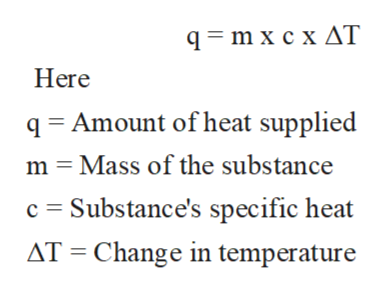# When 0.514 g of biphenyl (C12H10) undergoes combustion in a bomb calorimeter, the temperature rises from 25.0 ∘C to 29.9 ∘C.Find ΔErxn for the combustion of biphenyl. The heat capacity of the bomb calorimeter, determined in a separate experiment, is 5.86 kJ/∘C.Express the energy in kilojoules per mole to three significant figures.

Question
200 views

When 0.514 g of biphenyl (C12H10) undergoes combustion in a bomb calorimeter, the temperature rises from 25.0 ∘C to 29.9 ∘C.

Find ΔErxn for the combustion of biphenyl. The heat capacity of the bomb calorimeter, determined in a separate experiment, is 5.86 kJ/∘C.

Express the energy in kilojoules per mole to three significant figures.
check_circle

Step 1

The specific heat can be defined as the amount of heat per unit mass that is needed to raise the temperature of given substance by one degree Celsius.

The specific heat of water is 4.186 joule/gram °C. This can be used to calculate the heat required to raise the temperature for given substance.

Step 2

The relation between heat change and specific heat is shown below;help_outlineImage Transcriptionclose93 mxсхдT Here q Amount of heat supplied m Mass of the substance c Substance's specific heat Change in temperature AT fullscreen
Step 3

Heat capacity of the bomb calorimeter= 5.86 kJ/∘C

Tf = final temperature = 29.9 ∘C

Ti = Initial temperature = 25.0 ∘C...

### Want to see the full answer?

See Solution

#### Want to see this answer and more?

Solutions are written by subject experts who are available 24/7. Questions are typically answered within 1 hour.*

See Solution
*Response times may vary by subject and question.
Tagged in

### Equilibrium Concepts